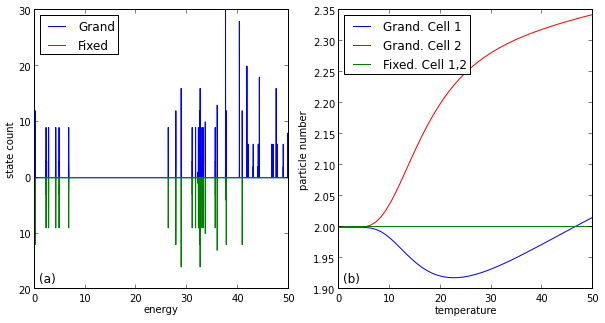# Fixed Charge approximation¶

This notebook briefly compares the fixed charge approximation to the exact, grand canonical system. For the fixed charge approximation we rescrict the Hilbert space to states where there are exactly two electrons (more generally, a fixed number of electrons) per cell. The chemical potential is zero, $\mu = 0$. Hence the approximation is valid as long as there actually are two electrons on each cell in the grand canonical system and as long as the energetically next highest charge sectors are sufficiently gapped (compared with the temperature).

Below we plot, for a two cell system, the energy histogram and the particle number per cell over temperature. The fixed charge system indeed reproduces the low energy part of the spectrum exactly. The particle numbers agree, as long as the temperature ($T < 10$) is much smaller than the energy gap ($\Delta E \sim 30$)

In :
%matplotlib inline
import matplotlib.pyplot as plt
import numpy as np
import qca
print(qca.__version__)

8.2.0

In :
s_g = qca.QcaGrandCanonical()
s_f = qca.QcaFixedCharge()

# Wire geometry
N = 2
V1 = 100.0
boa = 2.0
P_0 = 1

# Other parameters
V_0 = 1000
mu=250

# Set the systems up
s_f.l = qca.Wire(N,V1,boa,P_0)
s_g.l = qca.Wire(N,V1,boa,P_0)
s_f.V_0 = V_0
s_g.V_0 = V_0
s_f.mu = mu
s_g.mu = mu

# Takes about half an hour!
s_f.init()
s_f.run()
s_g.init()
s_g.run()

In :
# Are we in the right charge sector?
T = 1.0
s_g.T = T
s_f.T = T
s_g.run(True)
s_f.run(True)
print('Particle number for cell 1 and 2 in the grand canonical system: {:.2f}, {:.2f}'
.format(s_g.results['N'],s_g.results['N']))

Particle number for cell 1 and 2 in the grand canonical system: 2.00, 2.00

In :
# Create and plot an energy histogram
Es_g = np.array(s_g.energies())
Es_f = np.array(s_f.energies())
Emin = np.concatenate((Es_g, Es_f)).min()
Es_g -= Emin
Es_f -= Emin
Emin = 0
#Emax = np.concatenate((Es_g, Es_f)).max()

Emax = 100
bins = np.linspace(Emin,Emax,10000)
h_f,_ = np.histogram(Es_f,bins)
h_g,_ = np.histogram(Es_g,bins)
bins = bins[:-1]

# Particle number over temperature
Ns_g = []
Ns_f = []
for T in np.linspace(0.1,50,50):
s_g.T = T
s_f.T = T
s_g.run(True)
s_f.run(True)
Ns_g.append([T, s_g.results['N'],s_g.results['N']])
Ns_f.append([T, s_f.results['N'],s_f.results['N']])
Ns_g = np.array(Ns_g)
Ns_f = np.array(Ns_f)

---------------------------------------------------------------------------
NameError                                 Traceback (most recent call last)
<ipython-input-5-cddbf0a132fe> in <module>()
23     s_f.run(True)
24     Ns_g.append([T, s_g.results['N'],s_g.results['N']])
---> 25     Ns_f.append([T, s_fresults['N'],s_f.results['N']])
26 Ns_g = np.array(Ns_g)
27 Ns_f = np.array(Ns_f)

NameError: name 's_fresults' is not defined
In :
fig = plt.figure(figsize=(10,5))
p.plot(bins,h_g,'-',label='Grand')
p.plot(bins,-h_f,'-',label='Fixed')
p.set_xlim(0,50)
p.set_ylim(-20,30)
p.set_xlabel('energy')
p.set_ylabel('state count')
p.legend(loc='upper left')
p.get_yaxis().set_ticklabels([20,10,0,10,20,30])
p.text(0.02,0.02,'(a)',
fontsize='12',
horizontalalignment='left',
verticalalignment='bottom',
transform=p.transAxes)

p.plot(Ns_g[:,0],Ns_g[:,1],label='Grand. Cell 1',color='blue')
p.plot(Ns_g[:,0],Ns_g[:,2],label='Grand. Cell 2',color='red')
p.plot(Ns_f[:,0],Ns_f[:,1],label='Fixed. Cell 1,2',color='green')
p.set_xlabel('temperature')
p.set_ylabel('particle number')
p.legend(loc='upper left')
p.text(0.02,0.02,'(b)',
fontsize='12',
horizontalalignment='left',
verticalalignment='bottom',
transform=p.transAxes)

fig.savefig('../plots/chapter02/fixed_charge_approximation.pdf')In [ ]: